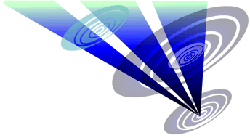Reconstruction of the 2021 Roundway Hill formation 1.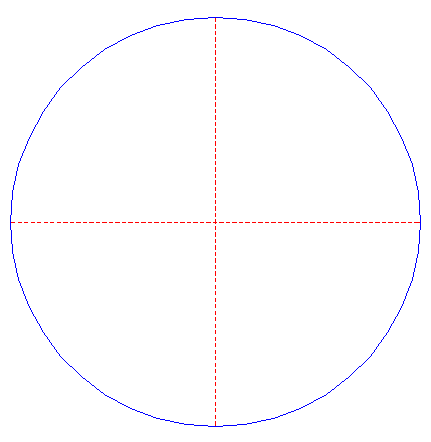Draw a circle. Draw the horizontal and vertical centerlines. 2.Construct the inscribed octagon (regular eight-sided polygon) of circle 1, pointing up. 3.Construct a "two-points" circle (defined by the two end-points of a centerline) between the top and the next angular point to the right of octagon 2. 4.Draw the connecting line between the centers of circles 1 and 3, and extend this line upto circle 1. 5.Construct the inscribed octagon of circle 1, with one angular point on line 4. 6.Draw sixteen rays of circle 1, from the center to the angular points of octagons 2 and 5. Number these rays (and angular points), clockwise, 1 - 16, starting at the top. 7.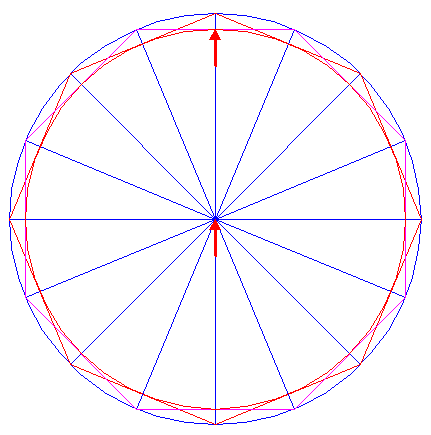Construct the inscribed circle of octagons 2 and 5. 8.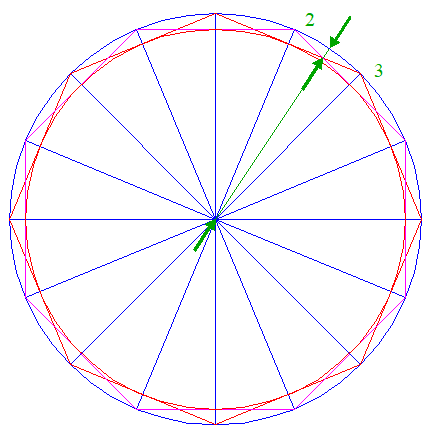Draw the connecting line between the center of circle 1 and the intersection of octagons 2 and 5 in between rays 6 nrs. 2 and 3, and extend this line upto circle 1. 9.Construct a circle centered at the top of octagon 2, passing through the intersection of circle 1 and line 8. 10.Copy circle 9 to the center of circle 1. 11.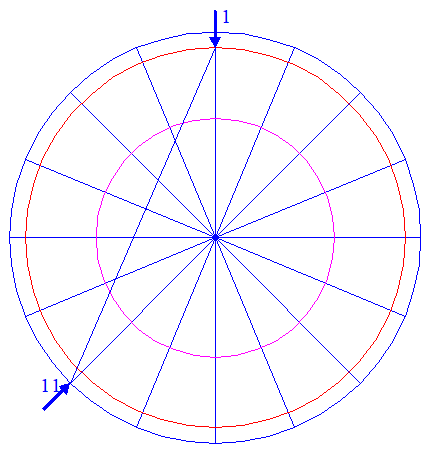Draw the connecting line between the intersection of circle 7 and ray 6 nr. 1, and angular point nr. 11. 12.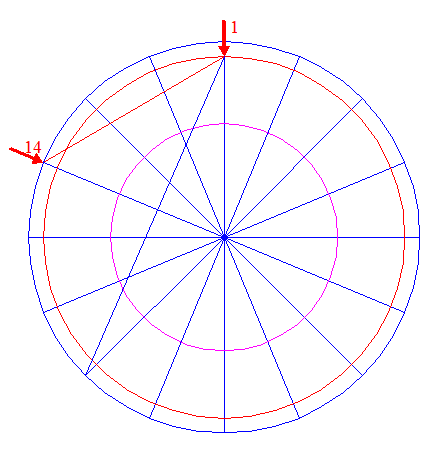Draw the connecting line between the intersection of circle 7 and ray 6 nr. 1, and angular point nr. 14. 13.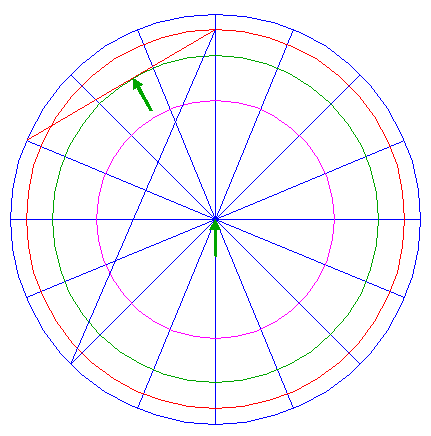Construct a circle concentric to circle 1, tangent to line 12. 14.Draw the connecting line between the intersection of circle 13 and ray 6 nr. 1, and angular point nr. 6. 15.Construct a "two-points" circle between the intersections of circles 1 and 7 with ray 6 nr. 1. 16.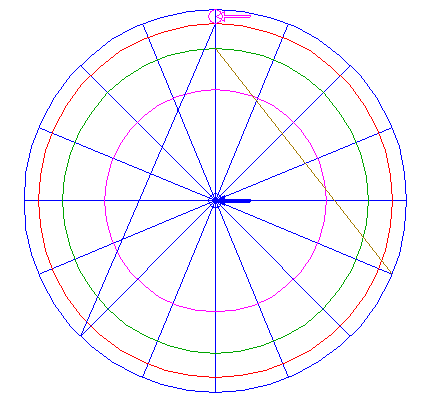Copy circle 15 to the center of circle 1. 17.Draw a line parallel to ray 6 nr. 1, tangent to circles 15 and 16 at the righthand sides. 18.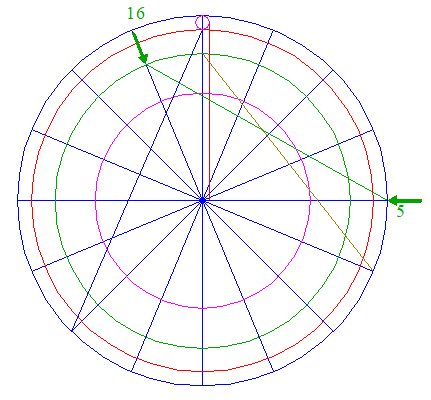Draw the connecting line between the intersection of circle 13 and ray 6 nr. 16, and angular point nr. 5. 19.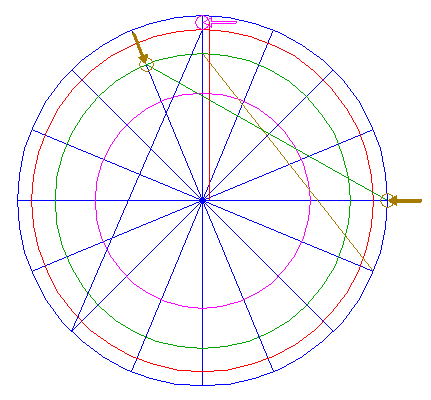Copy circle 15 two times, to the end-points of line 18. 20.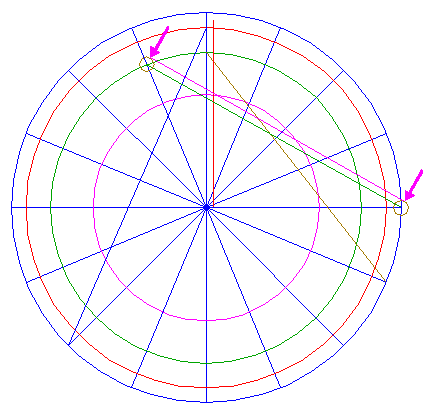Draw a line parallel to line 18, tangent to circles 19 at the upper sides. 21.Remove all redundant parts from circle 10, and lines 6, 11, 14, 17, and 20, such that... 22....this pattern remains. 23.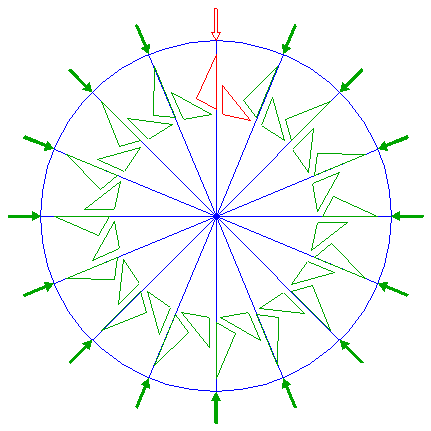Copy and rotate pattern 22 fifteen times, with respect to all other rays 6, as shown. 24.Construct a circle concentric to circle 1, tangent to line 11. 25.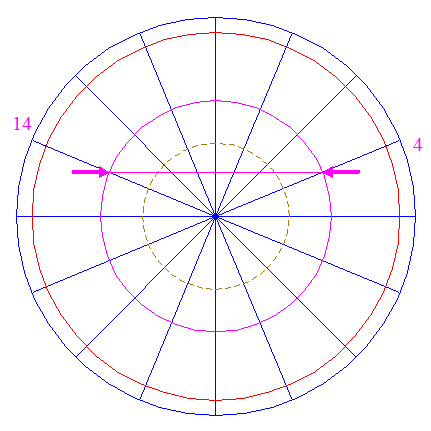Draw the connecting line between the intersections of circle 10 and rays 6 nrs. 4 and 14. 26.Construct a circle concentric to circle 1, tangent to line 25. 27.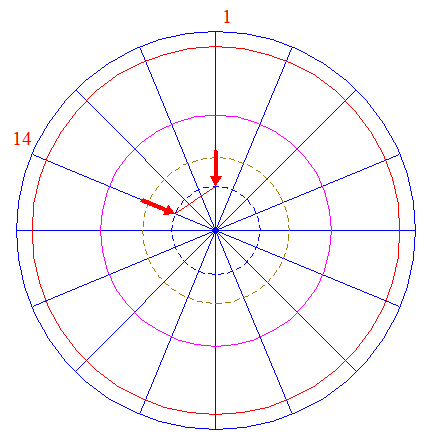Draw the connecting line between the intersections of circle 26 and rays 6 nrs. 1 and 14. 28.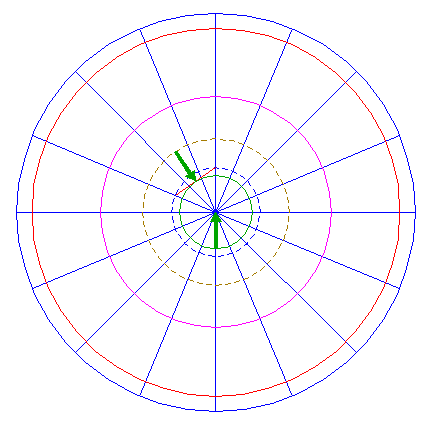Construct a circle concentric to circle 1, tangent to line 27. 29.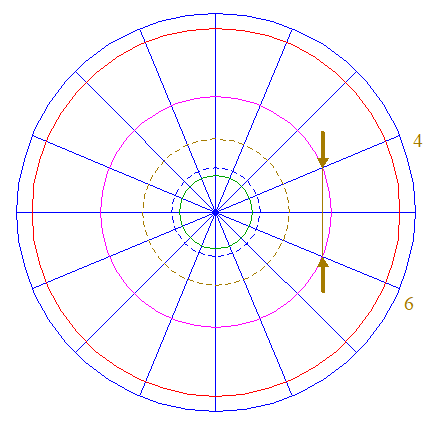Draw the connecting line between the intersections of circle 10 and rays 6 nrs. 4 and 6. 30.Construct a circle concentric to circle 1, tangent to line 29. 31.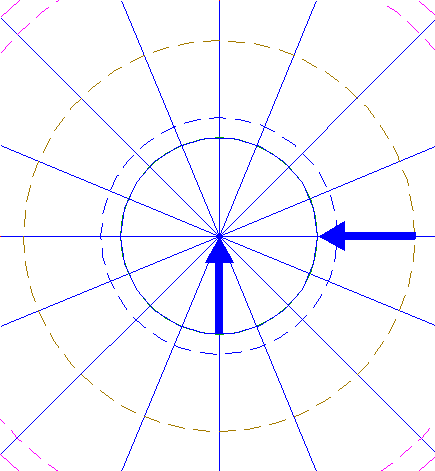Construct the inscribed regular 22-sided polygon of circle 28, pointing to the right. 32.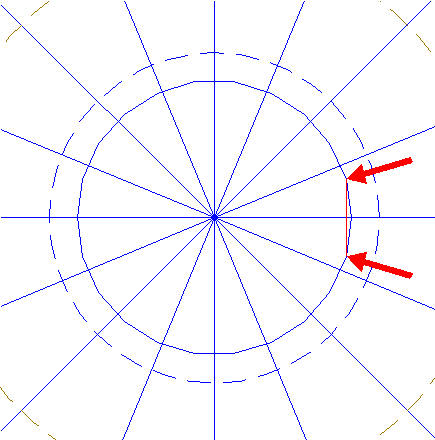Draw the connecting line between the upper and lower angular points of polygon 31, next to the rightmost one, as shown. 33.Construct a circle centered at the rightmost angular point of polygon 31, tangent to line 32. 34.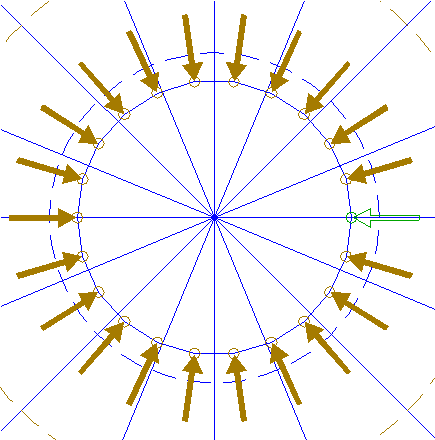Copy circle 33 twenty-one times, to the other angular points of polygon 31. 35.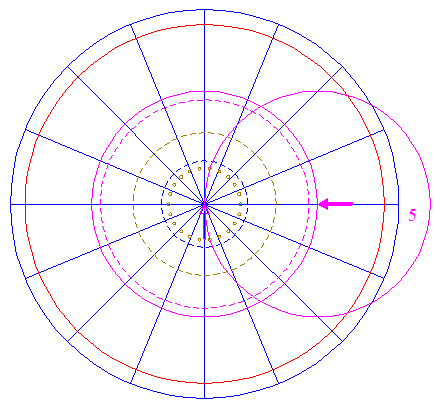Copy circle 10 to its intersection with ray 6 nr. 5. 36.Remove from circle 35 all redundant parts, such that... ...only this lower part in between circles 24 and 30 remains.37.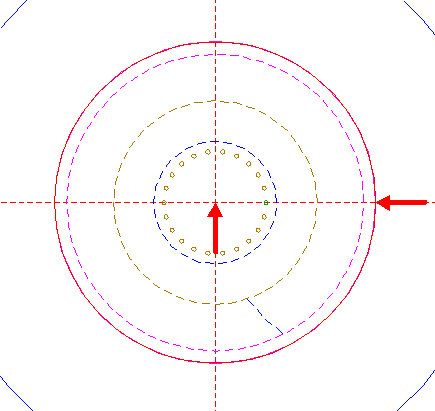Construct the inscribed regular 42-sided polygon of circle 10, pointing to the right. 38.Copy and rotate pattern 36 forty-one times, with respect to the other angular points of polygon 37, as shown. 39.Circles 1, 24, 26, 30, 33, and 34, and patterns 22, 23, 36, and 38, together, make up the final reconstruction. 40.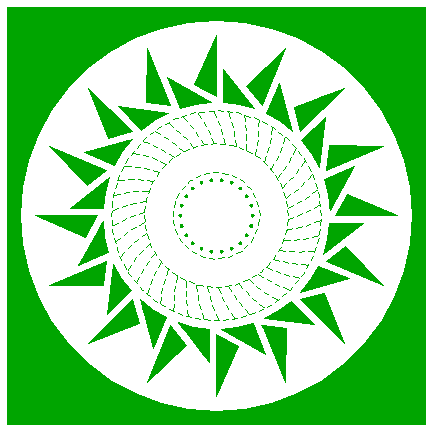Colour all areas corresponding to standing... 41.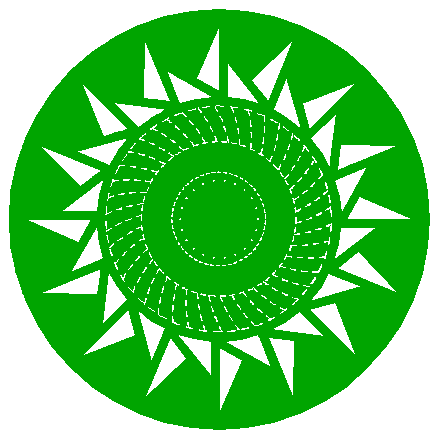...or to flattened crop, and finish the reconstruction of the 2021 Roundway Hill formation. 42.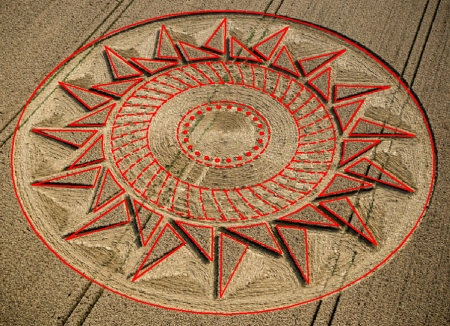The final result, matched with three aerial images.